# Harnack inequality

(dual Harnack inequality)

An inequality that gives an estimate from above and an estimate from below for the ratioof two values of a positive harmonic function; obtained by A. Harnack . Let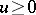be a harmonic function in a domainof an-dimensional Euclidean space; let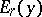be the ball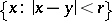with radiusand centre at the point. If the closure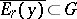, then the Harnack inequality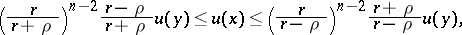(1)

or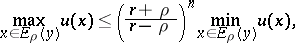is valid for all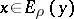,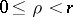. Ifis a compactum,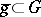, then there exists a number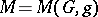such that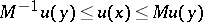(2)

for any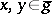. In particular,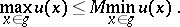Harnack's inequality has the following corollaries: the strong maximum principle, the Harnack theorem on sequences of harmonic functions, compactness theorems for families of harmonic functions, the Liouville theorem (cf. Liouville theorems), and other facts. Harnack's inequality can be generalized ,  to non-negative solutions of a wide class of linear elliptic equations of the form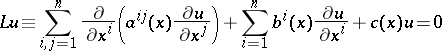with a uniformly positive-definite matrix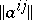: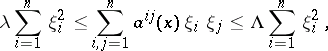where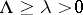are numbers,is any-dimensional vector and. The constantin inequality (2) depends only on,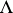, certain norms of the lower coefficients of the operator, and the distance between the boundaries ofand of.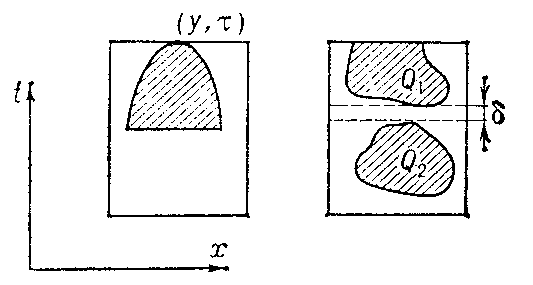Figure: h046600a

The analogue of Harnack's inequality is also applicable  to non-negative solutions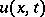of uniformly-parabolic equations of the form(the coefficients of the operatormay also depend on). In such a case only a one-sided inequality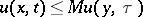is possible for points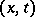lying inside the paraboloid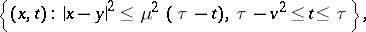which is concave downwards with apex at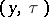(Fig., left part). Heredepends on,,,,,, on certain norms of the lower coefficients of the operator, and on the distance between the boundary of the paraboloid and the boundary of the domain on which. If, for instance,in the cylinderif the distance between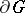and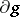is at least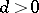and ifis sufficiently small, then the inequalityis valid in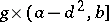. In particular, ifin(Fig., right part), if the compacta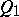and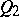are situated inand if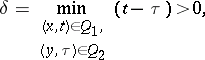thenwhere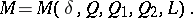The example of the function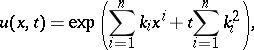which is a solution of the heat equation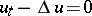for any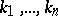, shows that in the parabolic case it is impossible to have two-sided estimates.